# Introduction

After working with diagrams for a while, you very quickly end up needing to manipulate points and vectors in order to position and describe your diagrams. For example, fromOffsets and fromVertices take lists of vectors and lists of points, respectively; beside and translate each take a vector as an argument; position expects objects paired with points; and so on.

This tutorial will walk you through everything you need to know about creating and working with vectors and points, with examples and exercises to deepen your understanding. If you notice any typos or bugs, are confused, or have an idea for extending or enhancing this tutorial, please open a ticket!

Solutions to the exercises can be found in the source code for this tutorial, in the diagrams-doc repository. Note, however, that many of the exercises have multiple good solutions.

# Vectors

Vectors in diagrams are based on the linear package. In two dimensions, you can think of a vector as a pair of coordinates, representing displacements in the $$x$$ and $$y$$ directions. Alternatively, you can think of a vector as consisting of a magnitude (length) and a direction (angle).One of the most important things to understand about vectors is that they are translation-invariant: that is, they have no specific location in space, and are unaffected by translations (though they are affected by other sorts of transformation such as scaling and rotation). You can see this for yourself at a ghci prompt:

>>> (3 ^& 6) :: V2 Double
V2 3.0 6.0
>>> translateX 19 (3 ^& 6) :: V2 Double
V2 3.0 6.0
>>> rotateBy (1/4) (3 ^& 6) :: V2 Double
V2 (-6.0) 3.0000000000000004

## Constructing vectors

Vectors in two dimensions have a type like V2 n, where n is some numeric type of scalar values (often Double). (One can also work with other vector spaces with any number of dimensions; in this tutorial we'll stick to the 2D case.)

The first thing to learn is how to create values of type V2 n. There are many options:

• zero is the zero vector, that is, the vector with zero magnitude (and no direction, or perhaps every direction). zero is rarely useful on its own, but can come in handy e.g. as an argument to a function expecting a vector input.

• unitX and unitY are the length-one vectors in the positive $$x$$ and $$y$$ directions, respectively. To create a length-$$l$$ vector you can apply scaling to unitX or unitY, like unitX # scale 3 or 3 *^ unitX (see Vector operations).

Also, unit_X and unit_Y are like unitX and unitY but point in the corresponding negative directions.> example = fromOffsets [unitX, unitY, 2 *^ unit_X, unit_Y] # centerXY
• To create a vector with given $$x$$- and $$y$$- components, you can use the function r2 :: (n, n) -> V2 n:> example = fromOffsets . map r2 $[(1,1), (0,3), (-2,1), (-1,-4)] As you can see, r2 is especially useful if you already have pairs representing vector components (which is not uncommon if the components are coming from some other data source). • You can also use the data constructor V2:> example = fromOffsets [V2 1 1, V2 0 3, V2 (-2) 1, V2 (-1) (-4)] • You can also use (^&) to construct vector literals, like so:> example = fromOffsets [1 ^& 1, 0 ^& 3, (-2) ^& 1, (-1) ^& (-4)] This can make for convenient and pleasant notation. However, it does have some drawbacks, namely: • (^&) is extremely general so its type is unhelpful. • Related to the above, literal vector expressions like 1 ^& 2 must be used in a context where the type can be inferred (or else a type annotation must be added). This is because (as we will see later) (^&) can also be used to construct points as well as higher-dimensional vectors. Only you can decide whether the tradeoffs are worth it in a given situation. • You can construct vectors from Directions using the fromDirection function. fromDirection takes a Direction and constructs a unit (i.e. magnitude 1) vector pointing in the given direction. • One final way to construct vectors is using the function e. By definition, e a == unitX # rotate a, but sometimes calling e can be more convenient. The name e is a sort of pun: in the same way that a complex number with magnitude $$r$$ and angle $$\theta$$ can be constructed as $$r e^{i\theta}$$, a vector with given magnitude and direction can be constructed as r *^ e theta. (Note that e is not exported from Diagrams.Prelude; if you wish to use it you must import it from Diagrams.TwoD.Vector.)> import Diagrams.TwoD.Vector > > example = lwG 0.05 . mconcat . map fromOffsets >$ [ [r *^ e (r @@ rad)]
>           | r <- [33 * tau/32, 34 * tau/32 .. 2 * tau]
>           ]

Construct each of the following images.

1.2. The circles have radius 1, and are arranged in the shape of a radius-5 semicircle.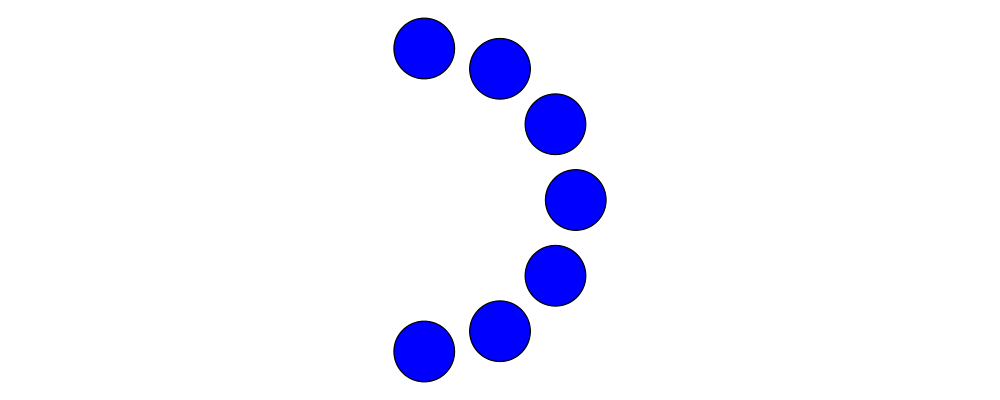3. 30 spokes with lengths 1, 2, and 3.## Destructing vectors

To take apart a vector into its $$x$$ and $$y$$ components, use unr2 :: V2 n -> (n, n), or more generally you can use coords (from Diagrams.Coordinates) and pattern-match on (:&). Both these methods work well in conjunction with the ViewPatterns GHC extension, as in

> foo :: V2 n -> ...
> foo (unr2 -> (x,y)) = ... x ... y ...

Note, however, that you will probably need this less often than you think. Using the vector operations presented in the next section, you should strive to work on the level of vectors, and only "stoop" to the level of working with explicit coordinates when absolutely necessary.

To get the magnitude and direction of a vector, you can use the norm and direction functions. To get the angle between two given vectors, use angleBetween. Additionally, quadrance gives the squared magnitude of a vector, and is more efficient than squaring the result of norm, since it avoids a call to sqrt. For example, if you want to test which of two vectors is longer, you can compare the results of quadrance instead of norm (since $$a < b \iff a^2 < b^2$$ as long as $$a$$ and $$b$$ are nonnegative).

## Vector operations

There is a rich set of combinators for operating on vectors (and we are open to adding more!).

• Vectors can be transformed with all the usual transformation functions like rotate, scale, and so on. However, recall that although it is possible to apply translate to a vector, it has no effect.> example = mconcat $map fromOffsets (map (:[]) vs) > where > vs = take 33 . iterate (scale (2**(1/32)) . rotateBy (1/32)) >$ unitX
• V2 is an instance of the Additive class (see Linear.Additive from the linear package). This means:

• Vectors can be added with (^+^). To add two vectors, think of placing them head-to-tail; the result of the addition is the vector from the tail of the first vector to the head of the second.

• There is a zero vector zero (mentioned previously), which is the identity for (^+^).

• Vectors can be negated with negated. The negation of a vector v is the vector with the same magnitude which points in the opposite direction, and is the additive inverse of v: that is, v ^+^ negated v == zero.

Linear.Additive also defines a few other methods which can be used on vectors, including (^-^) (vector subtraction) and sumV (summing an entire list or other Foldable container of vectors).

• V2 is also an instance of the Functor class (see Data.Functor from the base). The (*^) operator uses this class to multiply all components of a vector by a scalar. In particular for Num n => V2 n we have (*^) :: n -> V2 n -> V2 n. (Note that linear operators always use ^ in their names to indicate a vector argument, as in (*^) (scalar times vector) and (^+^) (vector plus vector) and (.+^) (point plus vector, as we will see later.)

Using (*^) is equivalent to using scale; that is, s *^ v == v # scale s. There is also a (^/) operator provided for convenience which divides a vector by a scalar; of course v ^/ s == v ^* (1/s).

• Finally, R2 is an instance of the Metric class (also in linear), which provides the inner product (also called dot product) function, dot. The definition and properties of the dot product are beyond the scope of this tutorial; you can read about it on Wikipedia. However, note that several common uses of the dot product are already encapsulated in other functions, such as project and leftTurn.

• The signorm function changes the magnitude of a vector to $$1$$, while keeping the direction fixed.

• perp yields a vector perpendicular to (and of the same magnitude as) its input.

• lerp linearly interpolates between two vectors as the given parameter varies from $$0$$ to $$1$$.

• leftTurn v1 v2 tests whether the direction of v2 is a "left turn" from v1 (that is, if the direction of v2 can be obtained from that of v1 by rotating up to one-half turn in the positive direction).

• project u v computes the projection of v onto u. In the illustration below, the green line shows the projection of the red vector onto the blue vector.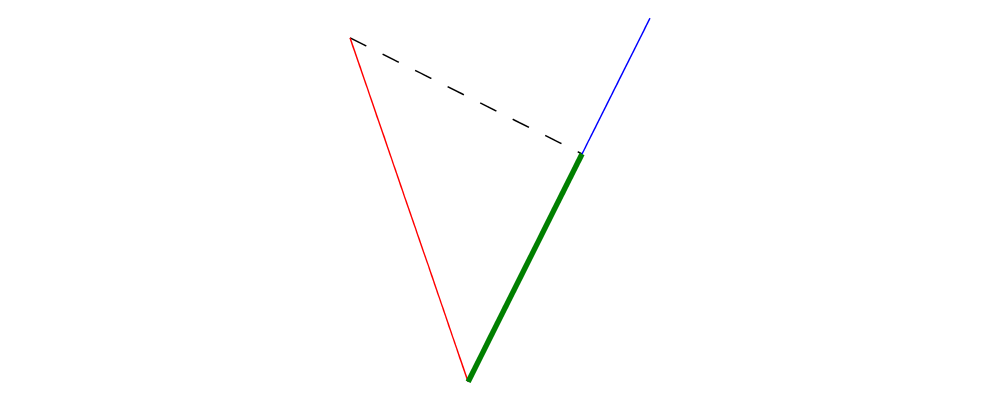> u = r2 (1,2)
> v = 2 *^ (unitY # rotateBy (1/19))
> p = project u v
>
> drawV v = fromOffsets [v]
>
> example = mconcat
>   [ drawV p # lc green # lwG 0.03
>   , drawV u # lc blue
>   , drawV v # lc red
>   , drawV (p ^-^ v) # translate v # dashingG [0.1,0.1] 0
>   ]
1. Write a function vTriangle :: V2 Double -> V2 Double -> Diagram B which takes as arguments two vectors representing two sides of a triangle and draws the corresponding triangle. For example, vTriangle unitX (unitX # rotateBy (1/8)) should produce2. Write a function which takes two vectors as input and constructs a classic illustration of vector addition using a parallelogram, as in the following example: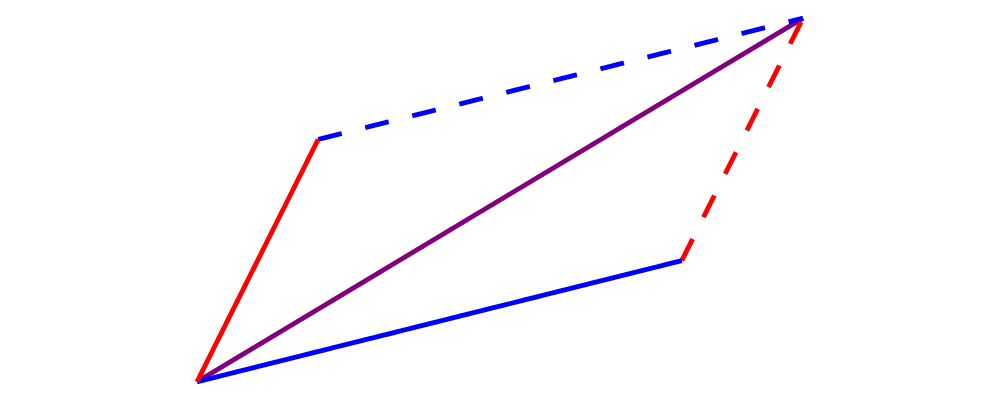## Using vectors

Once you have a vector, what can you do with it? A few of the things have already been seen in the examples above, but it's worth collecting a list here in one place.

• You can create a trail, path, or diagram (in fact, any TrailLike thing—see the trails and paths tutorial) from a list of vectors using fromOffsets.

• You can translate things by a vector using translate or moveOriginBy.

• As explained in the next section, you can add a vector to a point to yield another point.

# Points

A point is a location in space. In diagrams, points are based on the Point wrapper from the linear package, and in the case of 2D are represented by the type alias P2 = Point V2. In 2D, points are usually thought of as a pair of $$x$$ and $$y$$ coordinates (though other coordinate systems could be used as well, e.g. polar coordinates).

Points and vectors are closely related, and are sometimes conflated since both can be concretely represented by tuples of coordinates. However, they are distinct concepts which support different sets of operations. For example, points are affected by translation whereas vectors are not; two vectors can be added but two points cannot; and so on. Hence, they are represented by distinct types in diagrams.

## Constructing points

There are several ways to construct points.

• origin is the name of the distinguished point at the origin of the vector space (note this works in any dimension).

• To create a point with given $$x$$- and $$y$$- components, you can use the function p2 :: (n,n) -> Point V2 n: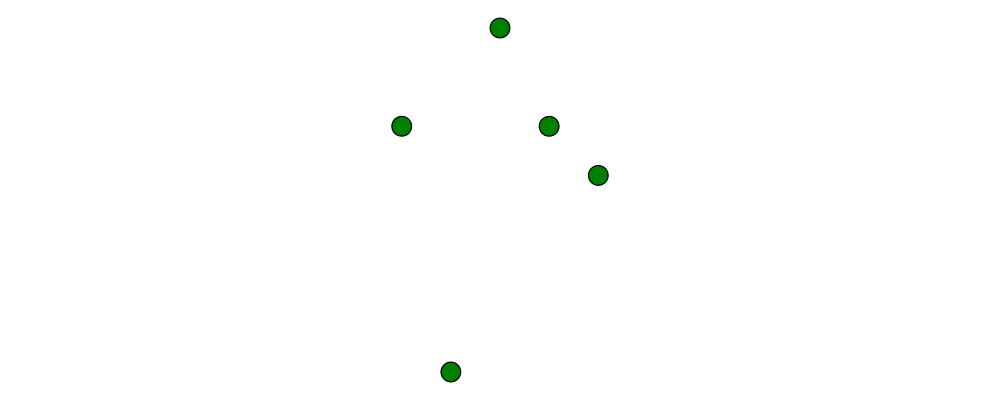> example
>   = flip atPoints (repeat (circle 0.2 # fc green))
>     $map p2$ [(1,1), (0,3), (-2,1), (-1,-4), (2,0)]

As with r2, p2 is especially useful if you already have pairs representing point coordinates.

• The ^& operator can be used to construct literal points (P2 n values) as well as vectors (V2 n values). The proper type is chosen via type inference: if the expression (3 ^& 5) is used in a context where its type is inferred to be P2 n, it is the point at $$(3,5)$$; if its type is inferred to be V2 n, it is the vector with $$x$$-component $$3$$ and $$y$$-component $$5$$.

• There is no way to directly convert a vector into a point (unless you use the P type constructor from Linear.Affine)—this is intentional! If you have a vector v and you want to refer to the point located at the vector's head (when the vector tail is placed at, say, the origin) you can write origin .+^ v (see below for a discussion of .+^).

• An advanced method of generating points is to use any function returning a TrailLike result, since [Point V2 Double] is an instace of TrailLike. Using a function returning any TrailLike at the result type [Point V2 Double] will result in the list of vertices of the trail. For example, here we obtain the list of vertices of a regular nonagon: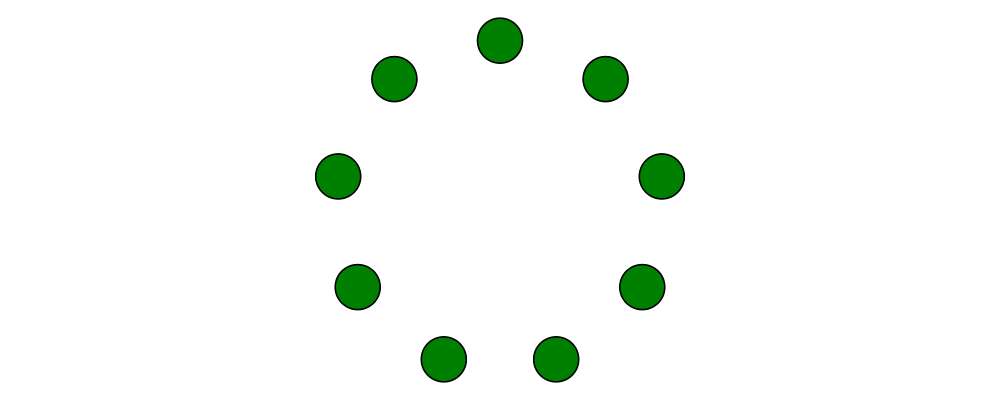> pts :: [P2 Double]
> pts = nonagon 1
> example = atPoints pts (repeat $circle 0.2 # fc green) Note that we could also inline pts in the above example to obtain > example = atPoints (nonagon 1) (repeat$ circle 0.2 # fc green)

In this case, the type of nonagon 1 would be inferred as [P2 Double] (since atPoints expects a list of points), causing the appropriate TrailLike instance to be chosen.

## Destructing points

For taking a point apart into its components:

• You can use the unp2 function, or, more generally, coords (just as with vectors) to get the Cartesian coordinates of a point.

• You can also use the _x and _y lenses to extract (or update) the $$x$$- and $$y$$-coordinates of a point: for example, pt ^. _x gets the $$x$$-coordinate of pt, and pt & _x +~ 2 adds 2 to the $$x$$-coordinate.

You can compute the distance between two points with the distance function (or qd to get the square ("quadrance") of the distance, which avoids a square root).

Construct each of the following images.

1. A $$31 \times 31$$ grid of circles, each colored according to the distance of its center from the origin.## Polar coordinates

Instead of being represented using $$x$$- and $$y$$-coordinates, points can also be represented using polar coordinates (usually referred to as $$(r, \theta)$$).

• You can use the _r lens to refer to the magnitude ($$r$$-coordinate) of a point.

• You can use the _theta lens to refer to the $$\theta$$-coordinate of a point, that is, the angle to the point as measured counterclockwise from the positive $$x$$-axis.

## Point operations

You can transform points arbitrarily: unlike vectors, points are affected by translation. Rotation and scaling act on points with respect to the origin (for example, scaling the point $$(1,1)$$ by $$2$$ results in the point $$(2,2)$$).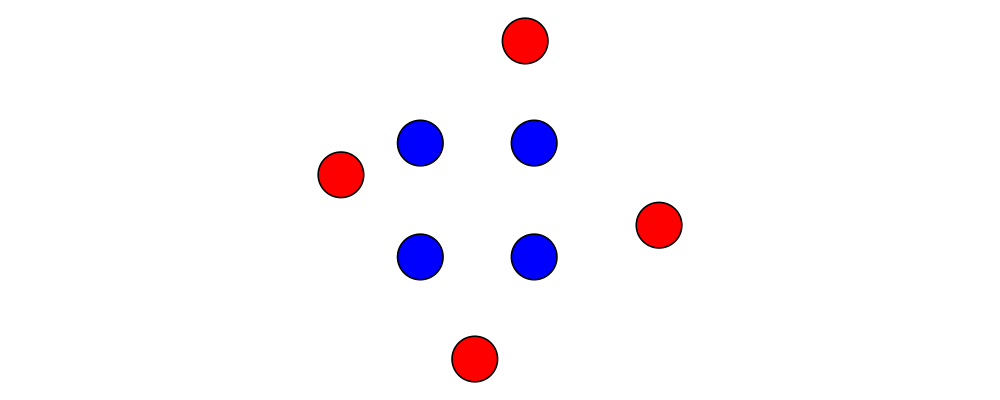> sqPts = square 1
>
> drawPts pts c = pts # map (\p -> (p,dot' c)) # position
> dot' c = circle 0.2 # fc c
>
> example = drawPts sqPts blue
>        <> drawPts (sqPts # scale 2 # rotateBy(1/10) # translateX 0.2) red

Abstractly, points and vectors together form what is termed an "affine space". Here is a nice intuitive description of affine spaces, stolen from the wikipedia page:

An affine space is what is left of a vector space after you've forgotten which point is the origin (or, in the words of the French mathematician Marcel Berger, "An affine space is nothing more than a vector space whose origin we try to forget about, by adding translations to the linear maps").

It's not important to understand the formal mathematical definition of an affine space; it's enough to understand the sorts of operations which this enables on points and vectors.

In particular, P2 is an instance of the Affine type class (defined in Linear.Affine from the linear package). This class also has an associated type family called Diff, which for P2 is defined to be V2: roughly, this says that the difference or "offset" between two points is given by a vector.

Note how the operators below are named: a period indicates a point argument, and a carat (^) indicates a vector argument. So, for example, (.+^) takes a point as its first argument and a vector as its second.

• You can "subtract" one point from another to get the vector between them, using (.-.). In particular b .-. a is the vector pointing from a to b.

• Using (.+^), you can add a vector to a point, resulting in another point which is offset from the first point by the given vector. If p .+^ v == p', then p' .-. p == v. You can also use (.-^) to subtract a vector from a point.

• Although it is not semanticly correct, Point is an instance of Additive (this may be fixed in a later release). This means you can linearly interpolate between two points using lerp, which does make sense. For example, to find the point which is 25% of the way from the first point to the second.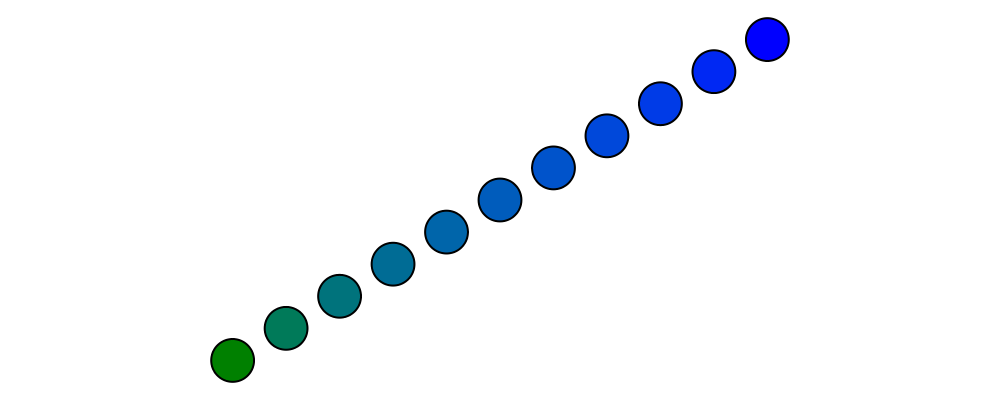> pt1, pt2 :: P2 Double
> pt1 = origin
> pt2 = p2 (5,3)
>
> example = position \$
>   [ (p, circle 0.2 # fc (colourConvert c))
>   | a <- [0, 0.1 .. 1]
>   , let p = lerp a pt2 pt1
>   , let c = blend a blue green
>   ]
• You can find the centroid (the "average" or "center of mass") of a list of points using the centroid function (defined in Diagrams.Points).

• Finally, you can scale a point using the (*^) operator (though, as mentioned earlier, you can also use scale).

1. Implement the Graham scan algorithm and generate diagrams illustrating the intermediate steps.

## Using points

Here are some things you can do with points, once you have constructed or computed them:

• You can create a straight line between two points with (~~).

• You can construct any TrailLike instance (like trails, paths, or diagrams) from a list of points using fromVertices.

• You can translate objects to a given point using moveTo, place, or moveOriginTo.

• You can position an entire collection of objects using position.